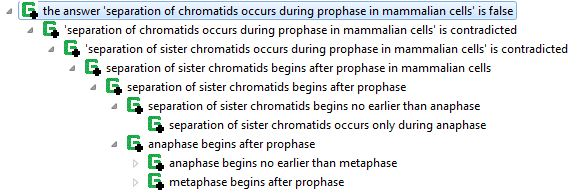# Pedagogical applications of proofs of answers to questions

In Vulcan’s Project Halo, we developed means of extracting the structure of logical proofs that answer advanced placement (AP) questions in biology.  For example, the following shows a proof that separation of chromatids occurs during prophase.This explanation was generated using capabilities of SILK built on those described in A SILK Graphical UI for Defeasible Reasoning, with a Biology Causal Process Example.  That paper gives more details on how the proof structures of questions answered in Project Sherlock are available for enhancing the suggested questions of Inquire (which is described in this post, which includes further references).  SILK justifications are produced using a number of higher-order axioms expressed using Flora‘s higher-order logic syntax, HiLog.  These meta rules determine which logical axioms can or do result in a literal.  (A literal is an positive or negative atomic formula, such as a fact, which can be true, false, or unknown.  Something is unknown if it is not proven as true or false.  For more details, you can read about the well-founded semantics, which is supported by XSB. Flora is implemented in XSB.)

### Now how does all this relate to pedagogy in future derivatives of electronic learning software or textbooks, such as Inquire?

Well, here’s a use case:

1. The electronic learning software or textbook asks user a multiple choice question
• (for the technically inclined, see the above referenced paper and this 3 year old video using SILK with knowledge translated from Aura, for example)
2. The student gets the question right or wrong.
• If the answer is wrong, the system can determine what could prove the incorrect answer given by the student as ask questions that involve those axioms in their proofs.
• As in Inquire, the student can quickly navigate to locations within the text that are pertinent to any given question by following the English sentences from which the axioms involved the proof were obtained.
• Also as in Inquire, the student can request a presentation of the ontology concerning the concepts and relationships between concepts involved in the proof (from any of which the student can similarly navigate to pertinent sections of the text).
• Note that here we are relying on the use of the Linguist to acquire the axioms from sentences in the text (or other sentences augmenting the text).  The sentences themselves can be presented in summary form, too.
• For each incorrect answer, the system can update its assessment of the student’s understanding of the concepts and relationships between those concepts, as well as the students comprehension of subject matter sentences so as to improve the pedagogical effectiveness of subsequently selected questions.  Alternatively, the system could be more aggressive to assess where comprehension is relatively weak rather than constructively engage a learner, as in pure assessment applications.
• If the answer is correct, the system can continue asking questions, perhaps preferring questions whose proofs involve axioms other than those involved in the correct answer.  Such questions might also be oriented to assessing how well the student understands axioms involved in proofs that other answers to the correctly answered question were proven wrong.
3. As the student answers questions correctly or incorrectly, the system assesses how well the student understands the underlying axioms and the vocabulary and ontological concepts used or referenced by those axiom
4. In one approach, the student can be asked questions in a bottom up manner.  That is, the student can be asked questions which involve axioms that reference concepts higher in the underlying ontology’s taxonomy.  As the student demonstrates understanding, the system can navigate the text or present sentences pertaining to slightly deeper or related material within the ontology.  For example, the questions Inquire suggests could be biased towards those that either assess by leaping deeper or teach by gradually moving from well comprehended subject matter to deeper or related content.